##Four-points

A four-point is a set of four points in general position {A,B,C,D}. This defines three more points {E,F,G} as intersections of the sides. By side we mean a line joining two points (out of the four). {E,F,G} are called diagonal points and the three lines {e,f,g} defined by pairs of them are the diagonals of the four-point.
Here we study the system of perspectivities naturally linked to a four-point.
 The (g,G)-perspectivity is the involutive homography S fixing the points of line g and the point G. Besides, each point I is mapped to J lying on line GI and such that {I,J} are harmonic conjugate to points G, GI, later being the intersection point of GI with g.
 The (f,F)-perspectivity is the involutive homography T fixing the points of line f and the point F. Besides, each point I is mapped to K lying on line FI and such that {I,K} are harmonic conjugate to points F, FI, later being the intersection point of FI with f.
 The two maps commute: S*T = T*S = R and define a third perspectivity, the (e,E)-perspectivity, fixing the points of line e etc..
 R2 = 1 is involutive, T = R*S = S*R and one could select two arbitrary from the set {R, S ,T} and prove the analogous properties for the third.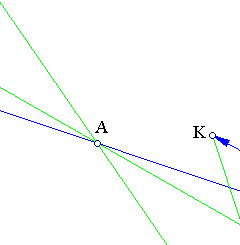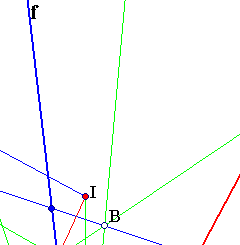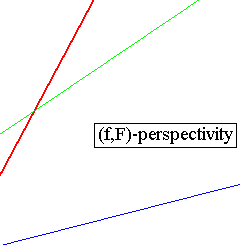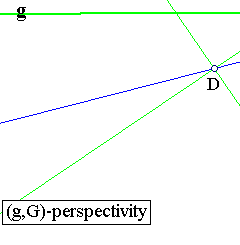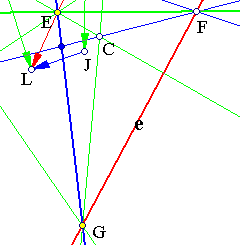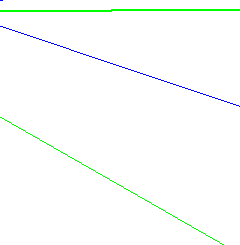The commutativity S*T = T*S generates for every point I of the plane another four-point and all these have the same diagonal points {E,F,G} and the same diagonals {e,f,g}. Considering the group generated by S and T, triangle EFG is its fundamental domain containing exactly one point of each orbit of the group action on the plane.
Considering the figure as part of the projective plane, triangle EFG defines four other triangles, each having a common side with the "central" one and a common vertex, the four triangles covering completely the plane. The maps {S, T, R} interchange these triangles with the "central" one.
Each of these triangles is also auto-polar with respect to every conic passing through the four points of an orbit of the group. In particular, when the triangle is obtuse there is a unique circle which is invariant with respect to the group. The involutions restricted on this circle become inversions (see Autopolar.html for a discussion of this circle).
See the file FourPointsCyclic.html for an illustration of the case of an obtuse triangle EFG and the corresponding unique, invariant under the group, circle.
In general the product of two involutions is a non involutive homography. The commutativity is equivalent with the condition that the Fregier point of each is contained in the homography axis of the other. This exactly is the case with the three involutions connected with a four-point.